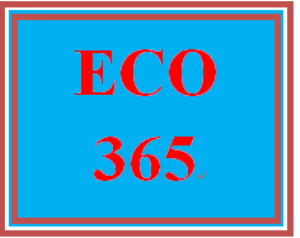# ECO 365 Week 3 Worksheet

PLDZ-7728 In Stock
\$ 8.00 USD
Description

ECO 365 Week 3 Worksheet

https://plus.google.com/u/0/108200033792883877670/posts/dWT4sDYbQKM

# ECO 365 Week 3 Worksheet

Complete the Worksheet.

Click the Assignment Files tab to submit your assignment.

Use the graph below to respond to (a) through (d):

1. a) If the firm represented by the above set of cost curves is able to sell its product in a perfectly competitive market for a price of \$5.50, it will maximize profit by producing how much output?

1. b) If the firm represented by the above set of cost curves is able to sell its product in a perfectly competitive market for a price of \$5.50 and it produces the profit-maximizing level of output, calculate the firm’s total revenue:

1. c) If the firm represented by the above set of cost curves is able to sell its product in a perfectly competitive market for a price of \$5.50 and it produces the profit-maximizing level of output, calculate the firm’s total cost:

1. d) If the firm represented by the above set of cost curves is able to sell its product in a perfectly competitive market for a price of \$5.50 and it produces the profit-maximizing level of output, calculate the firm’s profit:

Recent Reviews Write a Review
0 0 0 0 reviews